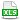HomeTechniques and Tips@RISK Distribution FittingBounds of Fitted Uniform and Exponential Distributions

# 4.13. Bounds of Fitted Uniform and Exponential Distributions

Applies to: @RISK 6.x/7.x, Professional and Industrial Editions

When I fit points to the continuous distributions RiskUniform and RiskExpon, the minimum of the fitted distribution is to the left of the smallest data value.  The maximum of the fitted RiskUniform is to the right of the largest data value.

This seems strange at first, but it actually makes good sense if you look deeper.  Here are two explanations:

• The data points you're fitting are a sample from some ideal theoretical distribution that you are trying to find. How likely is it, purely by chance, that your sample data points would include both the absolute minimum and the absolute maximum of the theoretical distribution? Extremely UNlikely. Therefore, the boundaries of the theoretical distribution are almost certainly wider than the boundaries of your sample data.

This issue is the famous German Tank Problem: given serial numbers of captured or destroyed tanks, how do you estimate the number of tanks that are being produced?

• More formally, consider the sampling distribution of an order statistic, in this case the minimum and possibly the maximum. Let n be the number of points in your sample. If you take many, many samples of size n from the theoretical distribution, and take the minimum of each sample, you have the sampling distribution of the minimum. The mean of that distribution (μmin) should equal the minimum of the data points. Some values in the sampling distribution will be above μmin and some will be below. The minimum (the left boundary) of the sampling distribution of the minimum must be less than μmin, which means it must be less than the minimum of the sample data.

With the uniform distribution, you can make an equivalent statement about the maximum. The exponential function is unbounded to the right, so there is no maximum.

@RISK lets you view a simulated sampling distribution of each parameter of each fitted distribution, such as the minimum and maximum of the fitted continuous uniform distribution. While setting up the fit, on the Bootstrap tab of the dialog, select Run Parametric Bootstrap. Then, on the Fit Results window, click the Bootstrap Analysis icon, which is the next to last one in the row at the bottom of the window. Select Parameter Confidence Intervals. Select a distribution at the left, select a parameter at the top, and see the graph of the simulated sampling distribution of that parameter. To see the statistics of the distribution, click the drop-down arrow at the top right of the graph and select Legend (with Statistics) or Statistics Grid.

This issue will come up in any bounded continuous distribution, where the probability density shifts abruptly at the left from zero to a positive value, or at the right from a positive value to zero.

For example, if you fit the points {11,12,13,14,15} as a continuous uniform distribution, you get RiskUniform(10,16), not RiskUniform(11,15) as you might expect at first. (Please see attached illustration.)  To make μmin and μmax equal the minimum and maximum of the sample data, @RISK applies a bias correction of (maxmin)/(n–1) = (15–11)/(5–1) = 1, so the minimum and maximum of the RiskUniform are 1 unit left and right of the minimum and maximum of the data.  For the points {11,11.5,12,12.5,13,13.5,14,14.5,15}, the bias correction is 0.5, and the fitted uniform function is RiskUniform(10.5,15.5).

For the exponential function, the bias correction is (meanmin)/n. Again considering the points {11,12,13,14,15}, the bias correction is (13–11)/5 = 0.4. (Please see attached illustration.)

Last edited: 2015-06-19

•FitComparison.xls